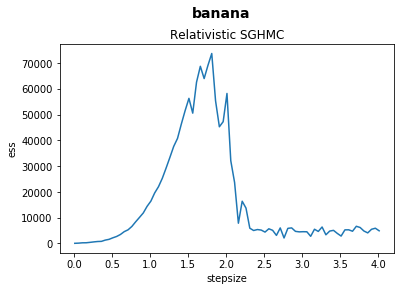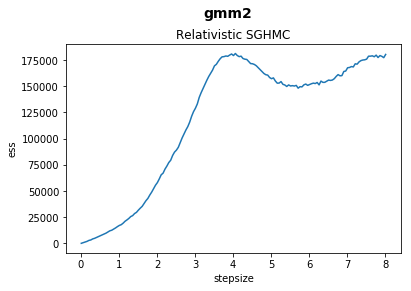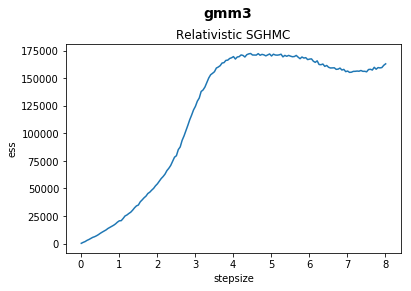# Effective Sample Sizes¶

In this notebook we explore and plot the relationship between stepsize and effective sample sizes of our samplers on various benchmark functions.

In :

%matplotlib inline
import matplotlib.pyplot as plt
import numpy as np

from string import capwords

import json
from os.path import abspath, basename, splitext
from glob import glob

samplers = (
"Relativistic_SGHMC", "SGHMC", "SGLD", "SVGD",
)

functions = (
"banana",
# "gmm1",   no data yet!
"gmm2",
"gmm3"
)

data_files = glob("{}/*".format(abspath("./data/effective_sample_sizes")))

for data_file in data_files:

sampler, file_extension = splitext(basename(data_file))
assert sampler in samplers
assert file_extension == ".json"

sampler = sampler.replace("_", " ")

with open(data_file, "r") as f:

for function in functions:
ess_data = sorted(data[function].items())

stepsizes = tuple(
stepsize for stepsize, _ in ess_data
)

average_ess = tuple(
np.mean(ess_values) for _, ess_values in ess_data
)

fig = plt.figure()
fig.suptitle(function, fontsize=14, fontweight="bold")